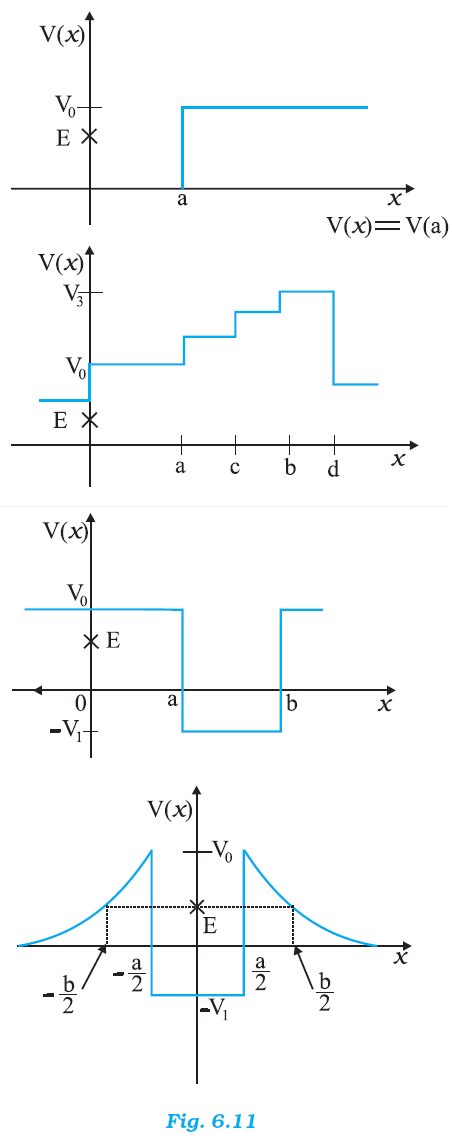## Pages

### Work, Energy and Power NCERT Solutions Class 11 Physics - Solved Exercise Question 6.3

Question 6.3:
Given in Fig. 6.11 are examples of some potential energy functions in one dimension. The total energy of the particle is indicated by a cross on the ordinate axis. In each case, specify the regions, if any, in which the particle cannot be found for the given energy. Also, indicate the minimum total energy the particle must have in each case. Think of simple physical contexts for which these potential energy shapes are relevant.Solution:
(a) x > a; 0
Total energy of a system is given by the relation:
E = P.E. + K. E.
∴ K.E. = E – P.E.
Kinetic energy of a body is a positive quantity. It cannot be negative. Therefore, the particle will not exist in a region where K.E. becomes negative.
In the given case, the potential energy (V0) of the particle becomes greater than total energy (E) for x > a. Hence, kinetic energy becomes negative in this region. Therefore, the particle will not exist is this region. The minimum total energy of the particle is zero.

(b) All regions
In the given case, the potential energy (V0) is greater than total energy (E) in all regions. Hence, the particle will not exist in this region.

(c) x > a and x < b;  –V1
In the given case, the condition regarding the positivity of K.E. is satisfied only in the region between x > a and x < b.
The minimum potential energy in this case is –V1. Therfore, K.E.  = E – (–V1) = E + V1. Therefore, for the positivity of the kinetic energy, the totaol energy of the particle must be greater than –V1. So, the minimum total energy the particle must have is –V1.

(d) -b/2 < x < a/2 ; a/2 < x < b/2 ; -V1

In the given case, the potential energy (V0) of the particle becomes greater than the total energy (E) for -b/2 < x < b/2 and -a/2 < x < a/2. Therefore, the particle will not exist in these regions.
The minimum potential energy in this case is –V1. Therfore, K.E.  = E – (–V1) = E + V1. Therefore, for the positivity of the kinetic energy, the totaol energy of the particle must be greater than –V1. So, the minimum total energy the particle must have is –V1.